fractal images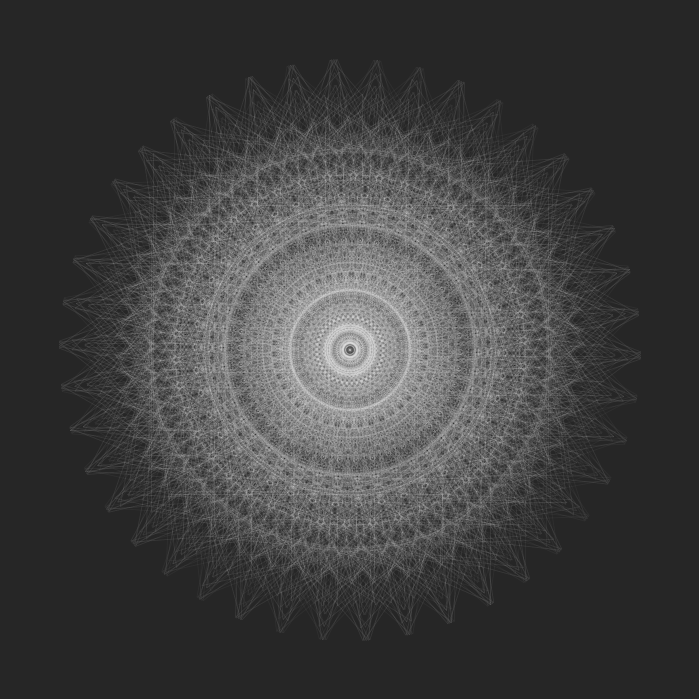$P=336,\alpha_0=210,\alpha_1=218.$

The recursive motif "F +60 F +240 F +60 F" produces the well-known Koch snowflake. Interestingly, for certain choices of angles, this recursive algorithm actually produces an image with finitely many segments and rotational symmetry.

The subdivision of the circle into 360 parts is arbitrary, however. The image above was produced by dividing the circle into 336 parts, and taking 210 and 218 of them, respectively, in the recursive algorithm, which would be described as "F +210 F +218 F +210 F." This is indicated in the caption, where the angles are called $\alpha_0$ and $\alpha_1$ — this is the notation I use in a paper I'm working on which discusses the mathematics of these images in detail. I'll post a link to it when I'm finished.

I stumbled on the white-on-gray color scheme as a result of including some of these images in a paper to be published. We were instructed to make sure that our images, if in color, looked good if printed in black and white. So I decided to use a white-on-gray motif, and was so pleased with the results that I stayed with it.

This is, for me, mathematical art at its purest. Even with the simplest of color schemes, the geometry of the images does all the work in generating the aesthetic appeal of the images.$P=336,\alpha_0=160,\alpha_1=168.$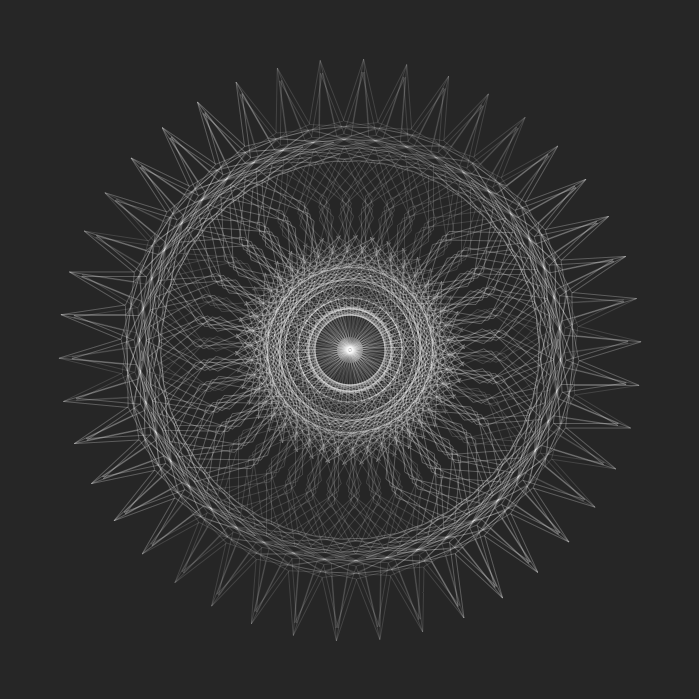$P=336,\alpha_0=53,\alpha_1=189.$$P=336,\alpha_0=97,\alpha_1=105.$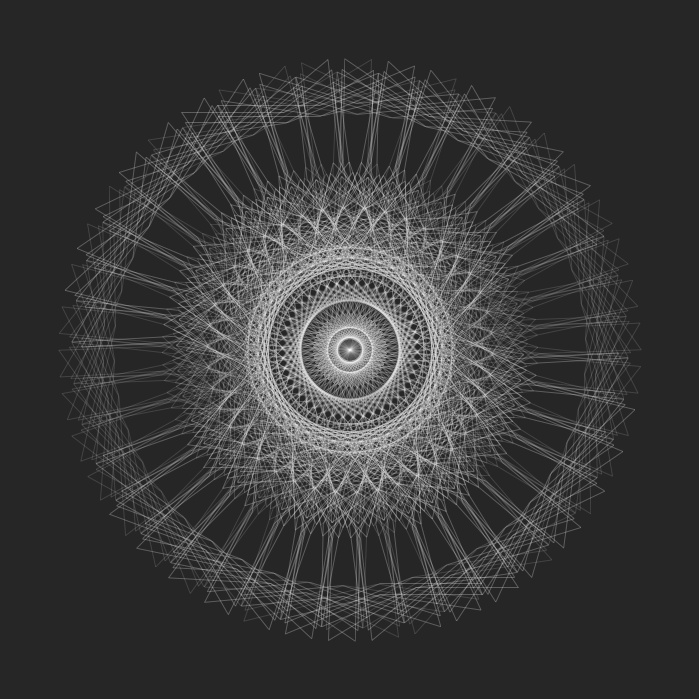$P=336,\alpha_0=115,\alpha_1=315.$$P=336,\alpha_0=134,\alpha_1=126.$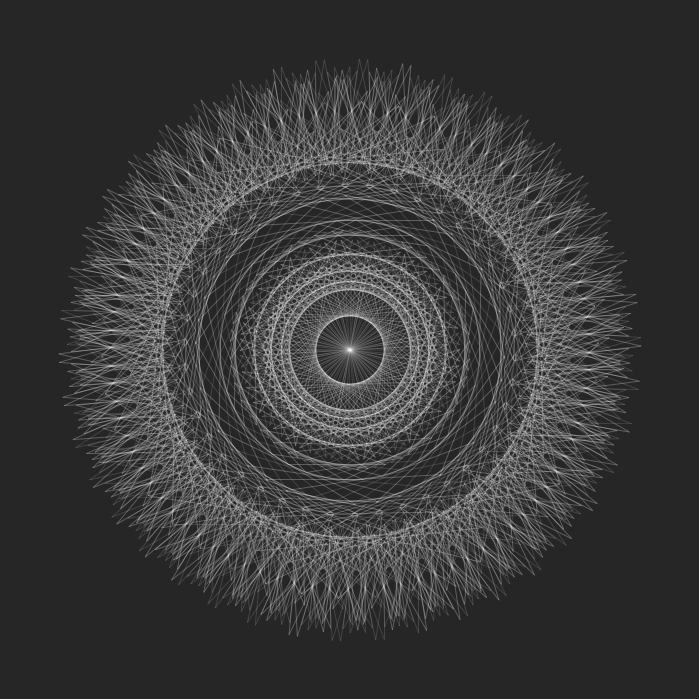$P=336,\alpha_0=149,\alpha_1=189.$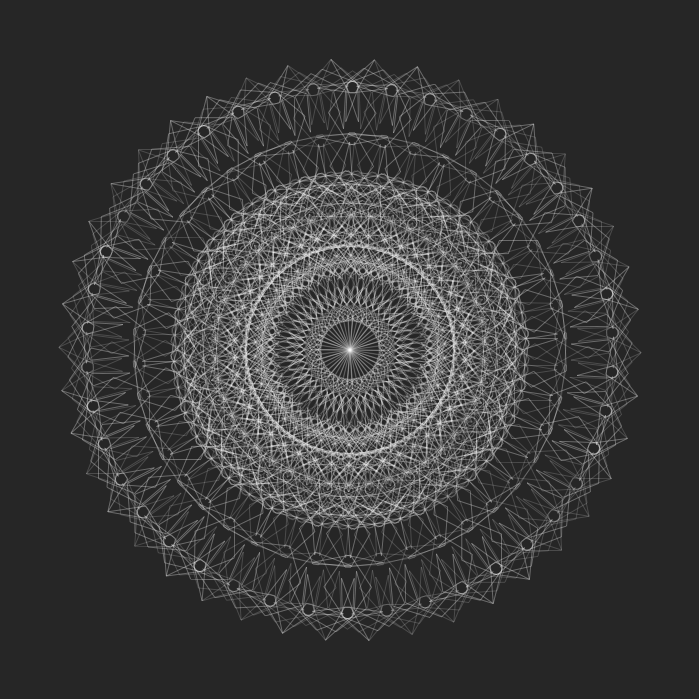$P=336,\alpha_0=273,\alpha_1=185.$

 © 2013-2016 vincent j matsko previous gallery next vince(dot)matsko(at)gmail(dot)com